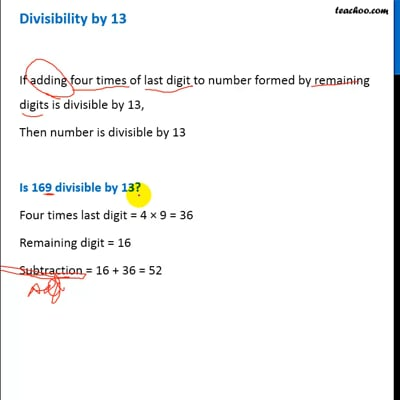Divisibility Tests - All

Chapter 3 Class 6 Playing with Numbers
Concept wise

If adding four times of last digit to number formed by remaining digits is divisible by 13,

Then number is divisible by 13

#### Is 169 divisible by 13?

Four times last digit = 4 × 9 = 36

Remaining digit = 16

Addition = 16 + 36 = 52

Since 52 is divisible by 13

∴ 169 is divisible by 13

#### Is 247 divisible by 13?

Four times last digit = 4 × 7 = 28

Remaining digit = 24

Addition = 24 + 28 = 52

Since 52 is divisible by 13

∴ 247 is divisible by 13

#### Is 298 divisible by 13?

Four times last digit = 4 × 8 = 32

Remaining digit = 29

Addition = 29 + 32 = 61

Since 61 is not divisible by 13

∴ 298 is not divisible by 13This video is only available for Teachoo black users